# DC Pandey Solutions: Motion in One Dimension- 3 Notes | Study DC Pandey Solutions for JEE Physics - JEE

## JEE: DC Pandey Solutions: Motion in One Dimension- 3 Notes | Study DC Pandey Solutions for JEE Physics - JEE

The document DC Pandey Solutions: Motion in One Dimension- 3 Notes | Study DC Pandey Solutions for JEE Physics - JEE is a part of the JEE Course DC Pandey Solutions for JEE Physics.
All you need of JEE at this link: JEE

Introductory Exercise 3.3

Ques 1: Figure shows the displacement-time graph of a particle moving in a straight line. Find the signs of velocity and acceleration of particle at time t = t1 and t = t2.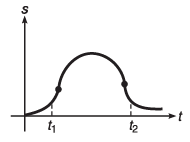Ans: vt1, at1 and at2 are positive while vt2 is negative
Sol: At t = t1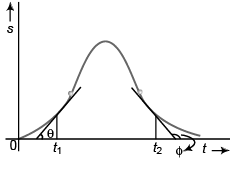v = tan θ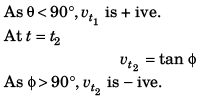Corresponding v-t graph will be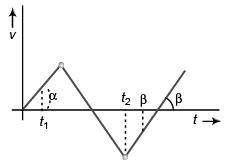Acceleration at t = t1 :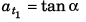As α < 90°, a t1 is + ive constant.
Acceleration at t = t2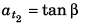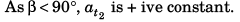Ques 2: A particle of mass m is released from a certain height h with zero initial velocity. It strikes the ground elastically (direction of its velocity is reversed but magnitude remains the same). Plot the graph between its kinetic energy and time till it returns to its initial position.
Ans: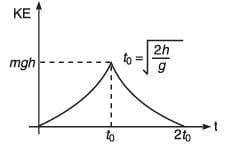Sol: Let the particle strike ground at time t velocity of particle when it touches ground

would be gt. KE of particle will be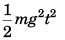i.e., KE ∝ t2. While going up the velocity will get - ive but the KE will remain. KE will reduce to zero at time 2 t when the particle reaches its initial position.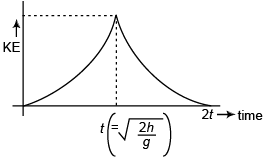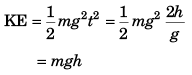Ques 3: A ball is dropped from a height of 80 m on a floor. At each collision, the ball loses half of its speed. Plot the speed-time graph and velocity-time graph of its motion till two collisions with the floor. [Take g = 10 m/s2]
Ans: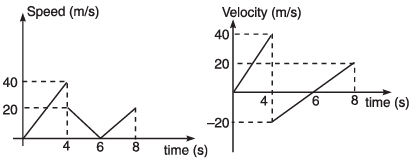Sol: Speed of ball (just before making first collision with floor)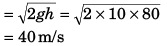Time taken to reach ground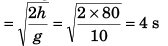Speed of ball (just after first collision with floor)
= 40/2 = 20 m/s
Time to attain maximum height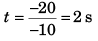∴ Time for the return journey to floor = 2 s.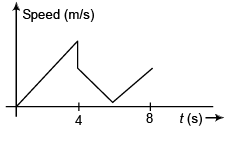Corresponding velocity-time will be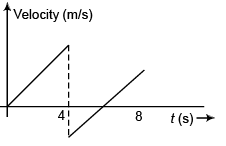Ques 4: Figure shows the acceleration-time graph of a particle moving along a straight line. After what time the particle acquires its initial velocity?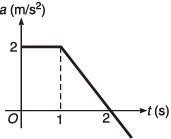Ans: (2 + √3) s
Sol: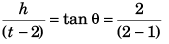⇒ h = 2 (t - 2)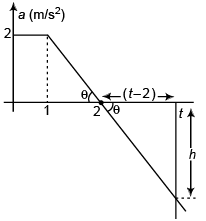Particle will attain its initial velocity i.e., net increase in velocity of the particle will be zero when,
area under a-t graph = 0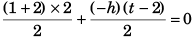or 3 - (t - 2)2 = 0
or (t - 2)2 = 3
or  t - 2 = ± √3
or   t = 2 ± √3
At time t = 2 + √3 s

Introductory Exercise 3.4

Ques 1: Two balls A and B are projected vertically upwards with different velocities. What is the relative acceleration between them?
Ans:
zero
Sol: Relative acceleration of A w.r.t. B
αAB = (+ g) - (+ g) = 0

Ques 2: In the above problem what is the shape of the graph between distance between the balls and time before either of the two collide with ground?
Ans: straight line passing through origin
Sol: Velocity of A w.r.t. B = vA - vB
∴ Relative displacement (i.e., distance between A  and B) would be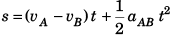or   s = (vA - vB) t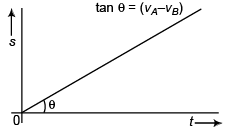Ques 3: A river 400 m wide is flowing at a rate of 2.0 m/s. A boat is sailing at a velocity of 10.0 m/s with respect to the water in a direction perpendicular to the river.
(a) Find the time taken by the boat to reach the opposite bank.
(b) How far from the point directly opposite to the starting point does the boat reach the opposite bank?
Ans: (a) 40 s
(b) 80 m
Sol: In figure, u = speed of boat
v = speed of river flow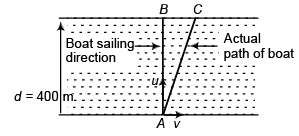Time to cross river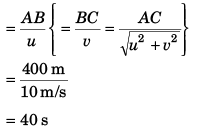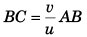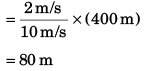Ques 4: An aeroplane has to go from a point A to another point B, 500 km away due 30° east of north. Wind is blowing due north at a speed of 20 m/s. The air-speed of the plane is 150 m/s.
(a) Find the direction in which the pilot should head the plane to reach the point B.
(b) Find the time taken by the plane to go from A to B.
Ans: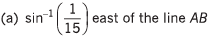(b) 50 min
Sol: Let C be the point along which pilot should head the plane.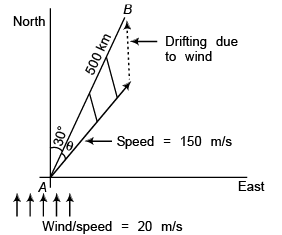Apply sine formula in Δ ABC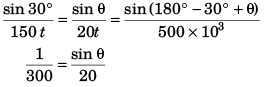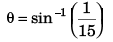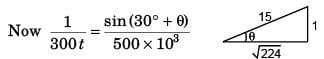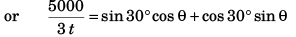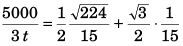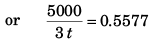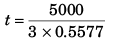= 2989 s
= 50 min

Ques 5: Two particles A and B start moving simultaneously along the line joining them in the same direction with acceleration of 1 m/s2 and 2 m/s2 and speeds 3 m/s and 1 m/s respectively. Initially A is 10 m behind B. What is the minimum distance between them?
Ans: 8 m
Sol: αA = 1 m/s2 ,   αB = 2 m/s2
vA = 3 m/s,   vB = 1 m/s
Acceleration of A w.r.t. B = 1 - 2 = - 1 m/s2
Velocity of A w.r.t. B = 3 - 1 = 2 m/s
Initial displacement of A w.r.t. B = - 10 m
At time relative displacement of A w.r.t. B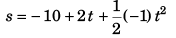or  s = - 10 + 2t - 0.5t2
For s to be minimum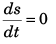or 2 - (0.5 x 2t) = 0
i.e., t = 2 s
∴ smin = - 10 + (2 x 2) - 0.5 x (2)2
= - 10 + 4 - 2
= - 8 m
Minimum distance between A and B = 8 m.

The document DC Pandey Solutions: Motion in One Dimension- 3 Notes | Study DC Pandey Solutions for JEE Physics - JEE is a part of the JEE Course DC Pandey Solutions for JEE Physics.
All you need of JEE at this link: JEEUse Code STAYHOME200 and get INR 200 additional OFF

## DC Pandey Solutions for JEE Physics

210 docs

Track your progress, build streaks, highlight & save important lessons and more!

,

,

,

,

,

,

,

,

,

,

,

,

,

,

,

,

,

,

,

,

,

;